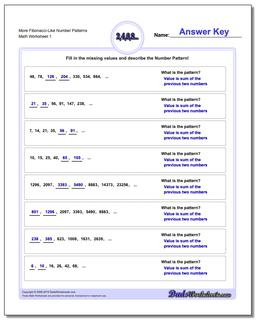# Math Worksheets: Number Patterns: Number Patterns: More Fibonacci-Like Number Patterns## More Fibonacci-Like Number Patterns

PropertyValue
DescriptionMore Fibonacci-Like Number Patterns: Fibonacci and Fibonacci-like number pattern problems, where the next values in a sequence are dependent on prior values.
Resource TypeWorksheet